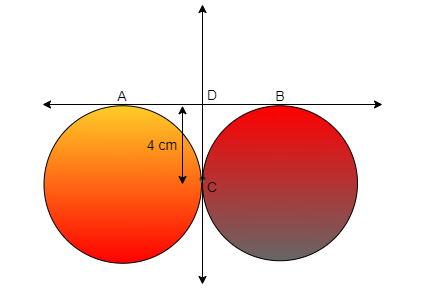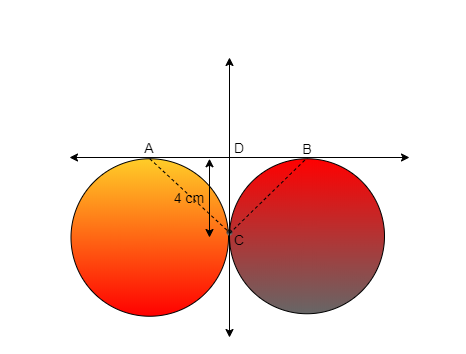QuestionAnswers

# AB and CD are the two common tangent to circles which touch each other at C. If D lies on AB such that CD = 4 cm, then AB is equal to A.4 cm B.6 cm C.8 cm D.12 cmHint: Before attempting this question remember to construct the diagram using the given information and use Point C is the common point that lie on the circumference of both the circles, use this information to approach towards the solution of the given question

Let’s construct the diagram using the given information
According to the given information we have two circles which touch each other at point C also the circle have 2 common tangents AB and CD
And there is a point D which lie on AB such that CD = 4cm
So the constructed diagram isTo find the value of AB let join Point B and C and point A and C so the diagram we get isAs we know that the two tangent drawn from the same external point from the circle are equal in length
Therefore we can say that CD = DB
Since CD = DB so DB = 4 cm
Similarly for tangent AD and DC by the property of tangent from the same external point form circle
Since AB is equal to AD + DB i.e. AB = AD + DB
Substituting the given values in the above equation we get
AB = 4 cm + 4 cm
AB = 8 cm
Since the measure of AB is 8 cm.
So, the correct answer is “Option C”.

Note: The concept of tangent played a main role to solve the above problem which can be explained as the line which intersect circle at one point that lie on the circumference of the circle here at the point where tangent intersect the circle, radius of the circle becomes perpendicular to the tangent. There are some basic properties that are shown by the tangent such as: the line of tangent never passes through the circle and the tangent drawn from the external points of the circle are equal to each other.
Tangent FormulaTo Determine the Mass of Two Different Objects Using a Beam BalanceWhat are the Successor and Predecessor?CBSE Class 10 Maths Chapter 10 - Circles FormulaWhat are the Functions of the Human Skeletal System?CBSE Class 9 Maths Chapter 6 - Lines and Angles FormulasCommon ColdCBSE Class 9 Maths Chapter 13 - Surface Areas and Volumes FormulasCBSE Class 10 Maths Chapter 8 - Introduction to Trigonometry FormulaCBSE Class 8 Maths Chapter 15 - Introduction to Graphs FormulasImportant Questions for CBSE Class 10 Maths Chapter 12 - Areas Related to CirclesImportant Questions for CBSE Class 9 Maths Chapter 10 - CirclesImportant Questions for CBSE Class 6 English A Pact with The Sun Chapter 9 - What Happened to The ReptilesImportant Questions for CBSE Class 9 Maths Chapter 5 - Introduction to Euclids GeometryImportant Questions for CBSE Class 9 Maths Chapter 4 - Linear Equations in Two VariablesImportant Questions for CBSE Class 6 English A Pact with The Sun Chapter 1 - A Tale of Two BirdsImportant Questions for CBSE Class 9 Maths Chapter 9 - Areas of Parallelograms and TrianglesCBSE Class 7 Science Fibre to Fabric WorksheetsCBSE Class 6 Science Fibre to Fabric WorksheetsImportant Questions for CBSE Class 10 Maths Chapter 10 - CirclesCBSE Class 12 Maths Question Paper 2020CBSE Class 10 Hindi A Question Paper 2020Hindi A Class 10 CBSE Question Paper 2009Hindi A Class 10 CBSE Question Paper 2015Hindi A Class 10 CBSE Question Paper 2007Hindi A Class 10 CBSE Question Paper 2013CBSE Class 10 Maths Question Paper 2020CBSE Class 10 Maths Question Paper 2017Hindi A Class 10 CBSE Question Paper 2016Hindi A Class 10 CBSE Question Paper 2012RD Sharma Solutions for Class 9 Maths Chapter 16 - CirclesNCERT Solutions for Class 9 Maths Chapter 10 CirclesNCERT Solutions for Class 10 Maths Chapter 12 Areas Related to CirclesRD Sharma Solutions for Class 10 Maths Chapter 15 - Areas Related to CirclesNCERT Solutions for Class 9 Maths Chapter 5 Introduction to Euclid’s GeometryRD Sharma Solutions for Class 9 Maths Chapter 7 - Introduction to Euclid's GeometryNCERT Solutions for Class 10 Maths Chapter 12 Areas Related to Circles in HindiNCERT Solutions for Class 9 Maths Chapter 4 Linear Equations in Two VariablesNCERT Solutions for Class 9 Maths Chapter 9 Areas of Parallelograms and TrianglesNCERT Exemplar for Class 10 Maths Chapter 11 - Areas Related to Circles (Book Solutions)